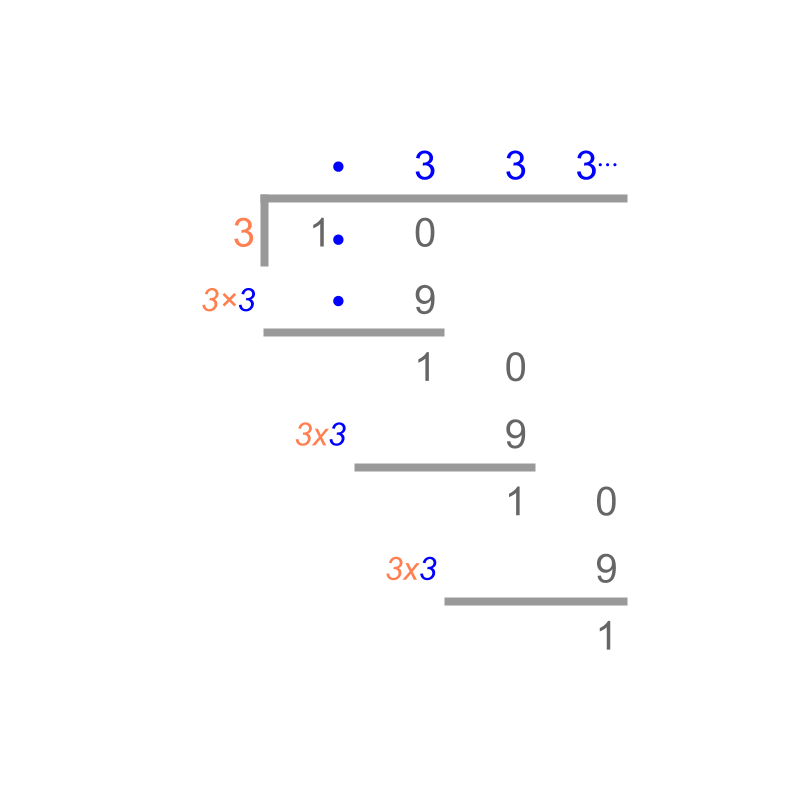maths > decimals

Conversion of Repeating Decimals to Fractions

what you'll learn...

overview

•  Some fractions result in decimals with repeating digits.

•  Representation of Repeating Decimals.

•  Conversion of Repeating Decimals to equivalent Fractions : A decimal has two parts, a non-repeating part at the beginning and repetitive part. With some simple arithmetics, equivalent fraction is derived.

eg: $0.\overline{3}$$0. \overline{3}$ is represented as $10x-x=3.\overline{3}-0.\overline{3}$$10 x - x = 3. \overline{3} - 0. \overline{3}$. The value of $x$$x$ is derived as fraction $\frac{1}{3}$$\frac{1}{3}$.

never ending...Convert $\frac{1}{3}$$\frac{1}{3}$ into a decimal. The answer is "$0.333\cdots$$0.333 \cdots$". It is noted that the decimal number does not end and number $3$$3$ repeats. The long-division method to convert the fraction into a decimal is illustrated in the figure.

Convert $\frac{23}{9}$$\frac{23}{9}$ into a decimal.
The answer is "$2.555\cdots$$2.555 \cdots$".
Note: $\cdots$$\cdots$ is used to represent that the digits are repeating.

Convert $\frac{371}{990}$$\frac{371}{990}$ into a decimal.
The answer is "$0.37474\cdots$$0.37474 \cdots$". It is noted that the decimal number does not end and number $7$$7$ and $4$$4$ repeats.

representing the repetition

$\frac{371}{990}=0.37474\cdots$$\frac{371}{990} = 0.37474 \cdots$

In the above representation with $\cdots$$\cdots$, it is not clear which part of the digits are repeating

•  is $4$$4$ repeated? like $0.37474444444\cdots$$0.37474444444 \cdots$

•  is $74$$74$ repeated? like $0.37474747474\cdots$$0.37474747474 \cdots$

•  is $37474$$37474$ repeated? like $0.3747437474\cdots$$0.3747437474 \cdots$

To avoid the confusion the following representation is adapted. The number is given as
$0.3\overline{74}$$0.3 \overline{74}$

The line over $74$$74$ represents that $74$$74$ is repeated.

Convert $\frac{1}{3}$$\frac{1}{3}$ into a fraction.
The answer is $0.\overline{3}$$0. \overline{3}$

Representation of Repeating Decimals : The repetitive pattern in decimal digits is represented with an over-line.

convert back to fraction

How do we convert $0.\overline{4}$$0. \overline{4}$ into a fraction?

To convert $0.\overline{4}$$0. \overline{4}$ into a fraction, the following steps are used

$x=0.\overline{4}$$x = 0. \overline{4}$
$10x=4.\overline{4}$$10 x = 4. \overline{4}$

Subtracting the two equations
$9x=4$$9 x = 4$
$x=\frac{4}{9}$$x = \frac{4}{9}$

examples

Converting $2.\overline{4}$$2. \overline{4}$ into a fraction.
$x=2.\overline{4}$$x = 2. \overline{4}$
$10x=24.\overline{4}$$10 x = 24. \overline{4}$

subtracting the two above
$9x=22$$9 x = 22$
$x=\frac{22}{9}$$x = \frac{22}{9}$
$x=2\frac{4}{9}$$x = 2 \frac{4}{9}$

To convert $2.23\overline{43}$$2.23 \overline{43}$ into a fraction:

$x=2.23\overline{43}$$x = 2.23 \overline{43}$
$100x=223.43\overline{43}$$100 x = 223.43 \overline{43}$
Subtracting the above,
$99x=221.20$$99 x = 221.20$
$990x=2212$$990 x = 2212$
$x=\frac{2212}{990}$$x = \frac{2212}{990}$.

Another method is as follows.
$100x=223+0.\overline{43}$$100 x = 223 + 0. \overline{43}$
$10000x=223×100+43.\overline{43}$$10000 x = 223 \times 100 + 43. \overline{43}$
Subtracting the two equations
$9900x=223×\left(100-1\right)+43$$9900 x = 223 \times \left(100 - 1\right) + 43$
$x=\frac{223}{100}+\frac{43}{9900}$$x = \frac{223}{100} + \frac{43}{9900}$

summary

Representation of Repeating Decimals : The repetitive pattern in decimal digits is represented with an over-line.

Conversion of Repeating Decimals to equivalent Fractions : A decimal has two parts, a non-repeating part at the beginning and repetitive part. With some simple arithmetics, equivalent fraction is derived.

eg: $0.\overline{3}$$0. \overline{3}$ is represented as $10x-x=3.\overline{3}-0.\overline{3}$$10 x - x = 3. \overline{3} - 0. \overline{3}$. The value of $x$$x$ is derived as fraction $\frac{1}{3}$$\frac{1}{3}$.

Outline